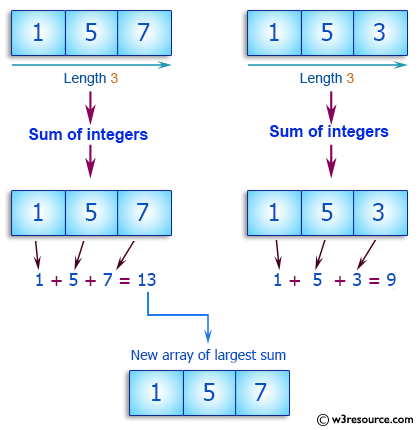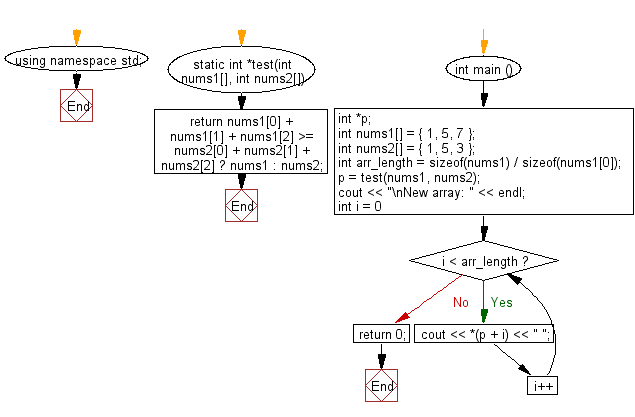﻿ C++ Exercises: Compute the sum of the two given arrays of integers, length 3 and find the array which has the largest sum - w3resource# C++ Exercises: Compute the sum of the two given arrays of integers, length 3 and find the array which has the largest sum

## C++ Basic Algorithm: Exercise-93 with Solution

Write a C++ program to compute the sum of the two given arrays of integers, length 3 and find the array which has the largest sum.

Sample Solution:

C++ Code :

``````#include <iostream>
using namespace std;

static int *test(int nums1[], int nums2[])
{
return nums1 + nums1 + nums1 >= nums2 + nums2 + nums2 ? nums1 : nums2;
}

int main () {
int *p;
int nums1[] = { 1, 5, 7 };
int nums2[] = { 1, 5, 3 };
int arr_length = sizeof(nums1) / sizeof(nums1);
p =  test(nums1, nums2);
cout << "\nNew array: " << endl;
for ( int i = 0; i < arr_length; i++ ) {
cout << *(p + i) << " ";
}
return 0;
}
``````

Sample Output:

```New array:
1 5 7
```

Pictorial Presentation:Flowchart:C++ Code Editor:

Contribute your code and comments through Disqus.

What is the difficulty level of this exercise?

﻿